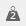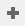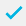# Using weights

A Weight may be used in several different ways:

• To create means in mean score tables
• To count items other than respondents, such as, amounts of money
• To change the proportions/balance of a sample group
• To increase/decrease the size of a sample group
• As part of a calculation

Multiple Response variables cannot be weighted and you cannot use more than one Weight in a table.

When tables have weights applied to them, and statistics produced, such as significances and confidence intervals, they are calculated using the weighted data not the unweighted.

## Defining a weight

Weights consist of a series of codes. They should have the same number of codes as the variable that you are applying the weight to.

1. Clickto display the Weights window.
2. Clickto add a new weight oron the Weights window toolbar to display the Weight Details dialog for an existing weight.

The image below shows a weight used to transform a five-code rating score into positive and negative values.

1. Enter a unique name in the Name field.
2. Enter a description of your weight in the Label field.
3. Enter the number of decimal places used in a calculation in the Decimal places field.
4. Enter the Value for each code in your weight. (Press Tab to create a new code). If you wish to exclude a variable code from the weighting, (e.g.”Don’t Know”) set its value to NA.
5. Clickto save the weight.
Contents